## Want to learn more how to use VLOOKUP to Sum Multiple Columns?

*** Watch our video and step by step guide below with free downloadable Excel workbook to practice ***

Watch on YouTube and give it a thumbs up 👍What does it do?

Searches for a value in the first column of a table array and returns the sum of values in the same row from other columns (to the right) in the table array.

Formula breakdown:

{=SUM(VLOOKUP(lookup_value, table_array, {col_index_num1,col_index_num2}, [range_lookup]))}

What it means:

{=SUM(VLOOKUP(this value, in this list, {and sum the value in this column, with the value in this column}, Exact Match/FALSE/0]))}

The VLOOKUP function can be combined with other functions such as the Sum, Max or Average to calculate values in multiple columns.  As this is an array formula, to make it work we simply need to press CTRL+SHIFT+ENTER at the end of the formula.  A very powerful feature for any serious analyst!

See how easy it is to implement in less than 1 minute with this VLOOKUP example!

We want to get the total number of units for Laptop (16,700 + 18,700 units).

STEP 1: We need to enter the VLOOKUP function in a blank cell:

## =VLOOKUP(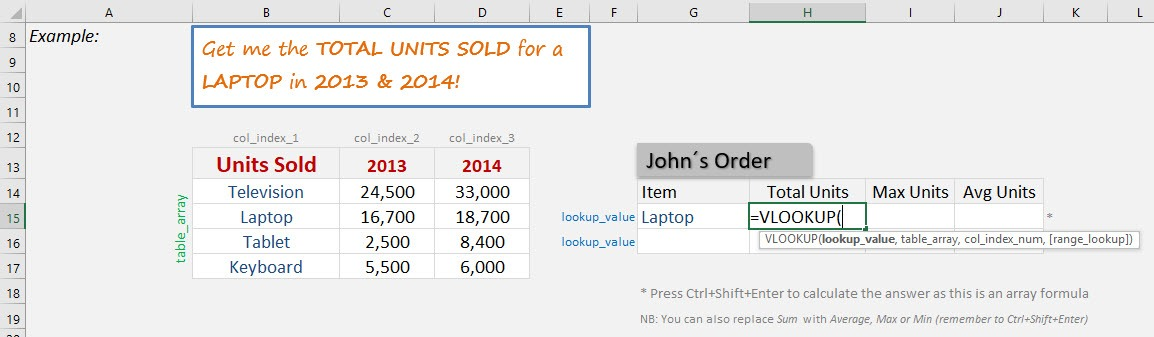STEP 2: The VLOOKUP arguments:

## Lookup_value

What is the value to be looked up?

Select the cell that contains the item name, which is Laptop.

## =VLOOKUP(G15,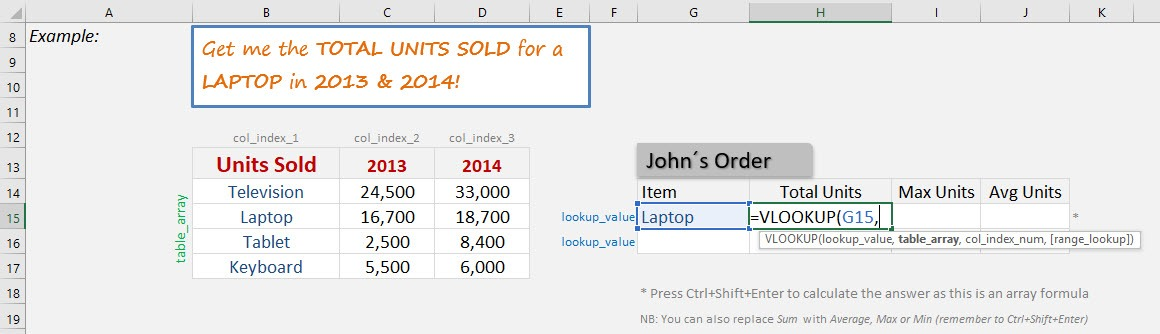## Table_array

Where is the list of data?

Select the Units Sold table, as that is where our formula is going to get the unit numbers.

## =VLOOKUP(G15, B14:D17,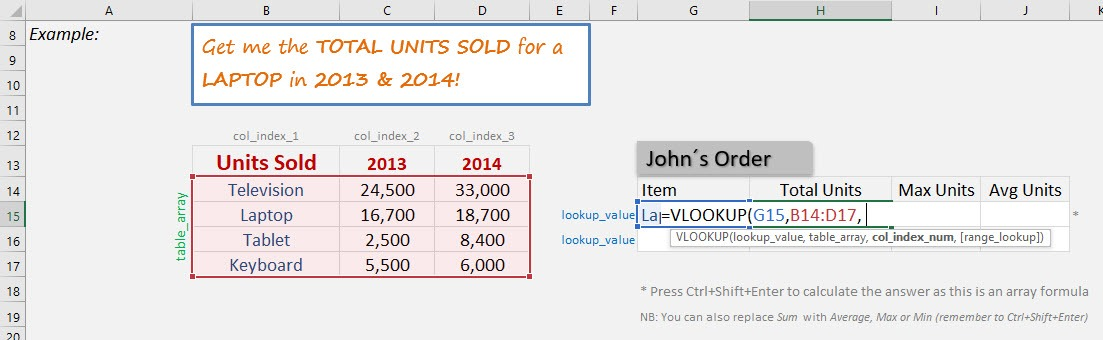## {Col_index_num1, Col_index_num2}

Which columns in the table_array contains the data you want to return?

We want to get the unit numbers of Years 2013 and 2014. So that will be columns 2 and 3.

## =VLOOKUP(G15, B14:D17, {2,3},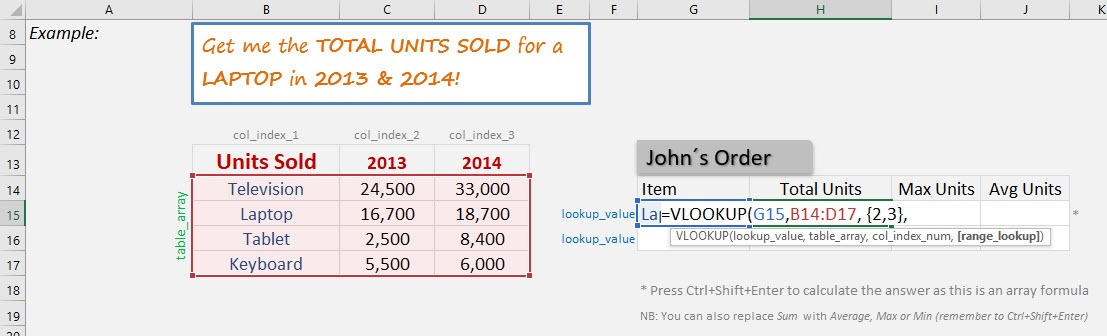## [Range_lookup]

Would it be an approximate match?

Set this to FALSE as we want an exact match for Laptop.

## =VLOOKUP(G15, B14:D17, {2,3}, FALSE)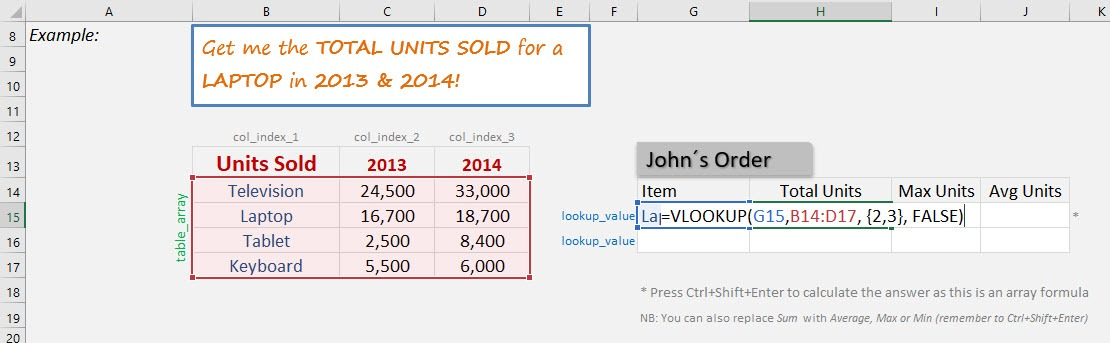STEP 3: Now wrap the formula with the SUM formula as we want to get the total number of sold units for Laptop.

## =SUM(VLOOKUP(G15, B14:D17, {2,3}, FALSE))

Ensure you are pressing CTRL+SHIFT+ENTER as we want to calculate this as an array formula.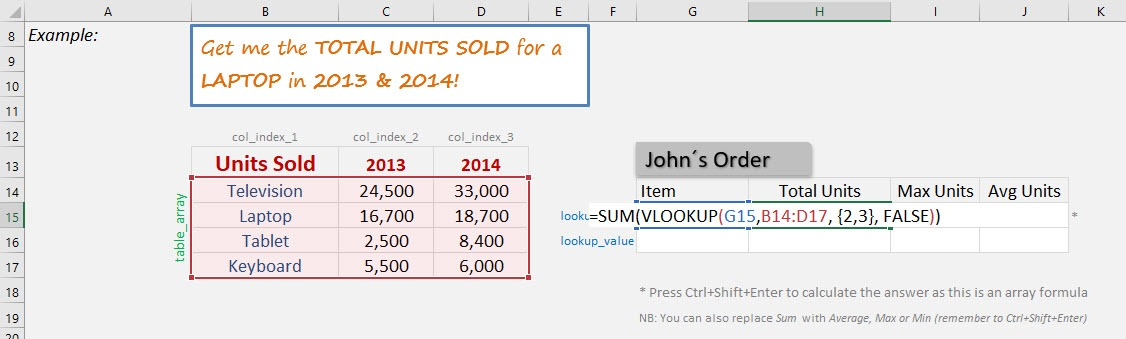Do the exact same formula for Max Units and Average Units, by changing the SUM Formula with the MAX Formula and Average Formula respectively.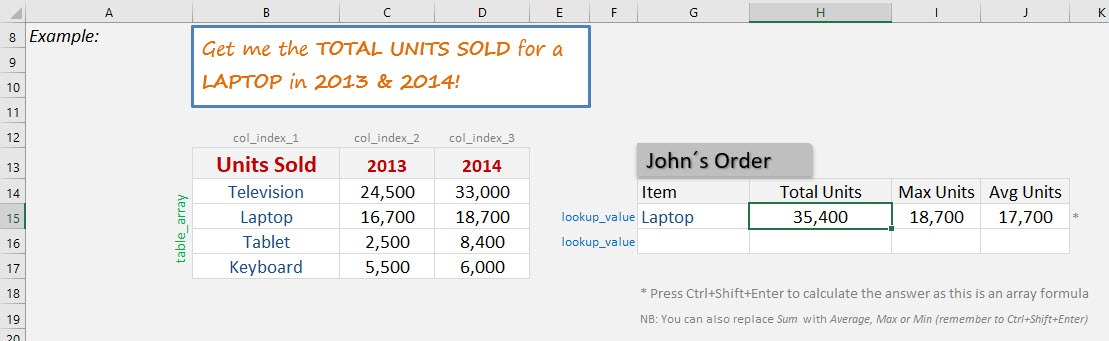How to VLOOKUP Multiple Columns in Excel### Related Excel Tutorials•Prasanta kumar Pannda says:

it’s won’t work for sum with vlookup. plz tell me how to take lookup value.
for my case it is taking only one column value not adding value.

thanks and regards,
Prasanta Kumar Panda

•Bryan says:

Hi Prasanta,

Could you share a screenshot or details of your worksheet? So that I can help understand the issue.

Thanks,
Bryan

•Sanjay says:

•John Michaloudis says:

Hey Sanjay,

Cheers,
John

•Joseph Mann says:

{=+MAX(VLOOKUP(\$G15,\$B\$14:\$D\$17,{2,3},FALSE))}

What is the purpose of the “{” and why do you put a “+” in front of the “Max” function?

•John Michaloudis says:

Hey Joseph,

The { means that the formula is an array formula. You can find what this means here: https://www.myexcelonline.com/excel-array-formulas-explained/

Regarding the + in front of a formula, I have a European keyboard and the + sign is easier to access than the = sign. The result is the same.

Cheers,
John

•Towhid says:

A good tool.

•John Michaloudis says:

•Aqib Siddique says:
•John Michaloudis says: